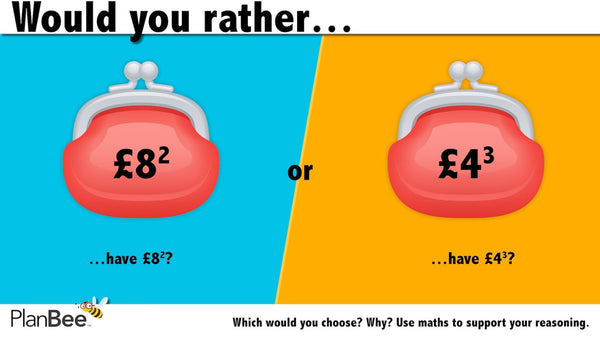# Would you rather…? Maths reasoning questions № 3

Here's another Maths reasoning question for KS1 or KS2 Maths lessons. It's ideal for use as a starter activity or as a puzzler to settle children as they arrive. It's the third in our new series of 'Would you rather…?' Maths reasoning questions.

These fun, visual problems are challenging, but open to interpretation by your pupils. For many of them, there is no 'right answer', but a choice of answers which children must first identify and choose from, then justify their choice using maths.

You'll find an explanation of the Maths involved in this 'Would you rather…?' Maths reasoning question directly underneath it, as well as links to all of the other questions in this problem-solving series.

## 'Would you rather…?' Maths reasoning question № 3:Would you rather have £8 squared or £4 cubed?

### Teacher's Notes

The 'solution':

8 x 8 = 64

4 x 4 x 4 = 64

Both purses contain the same amount.

You could challenge children to find the only other pair of smaller, whole square numbers and cube numbers that are equal (£4 squared and £2 cubed). Alternatively, challenge children to find other combinations of a square number and a cube number that have only a small difference between them, e.g. 5 cubed (75) and 9 squared (81). Who can find a combination with the smallest difference between them? \

Check out the other 'Would you rather…?' Maths reasoning questions.

Looking for more Maths Problem Solving lessons and plans? Check out our ready-to-teach collection here.